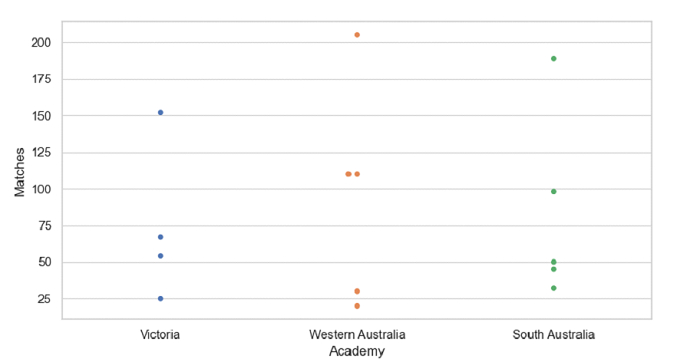# Python Pandas - Draw a swarm plot and control swarm order by passing an explicit order with Seaborn

PythonServer Side ProgrammingProgramming

Swarm Plot in Seaborn is used to draw a categorical scatterplot with non-overlapping points. The seaborn.swarmplot() is used for this. Control swarm order by passing an explicit order i.e. ordering on the basis of a specific column using the order parameter −

Let’s say the following is our dataset in the form of a CSV file −Cricketers2.csv

At first, import the required libraries −

import seaborn as sb
import pandas as pd
import matplotlib.pyplot as plt

Load data from a CSV file into a Pandas DataFrame −

dataFrame = pd.read_csv("C:\\Users\\amit_\\Desktop\\Cricketers2.csv")


Plotting swarm plot with Academy and Matches. Control swarm order by passing an explicit order i.e. ordering on the basis of "Academy" using order parameter −

sb.swarmplot(x = "Academy", y = "Matches", order = ["Victoria", "Western Australia", "South Australia"],

## Example

Following is the code

import seaborn as sb
import pandas as pd
import matplotlib.pyplot as plt

# Load data from a CSV file into a Pandas DataFrame

sb.set_theme(style="whitegrid")

# plotting swarm plot with Academy and Matches
# Control swarm, order by passing an explicit order i.e. ordering on the basis of "Academy" using order parameter
sb.swarmplot(x = "Academy", y = "Matches", order = ["Victoria", "Western Australia", "South Australia"],data= dataFrame)

# display
plt.show()

## Output

This will produce the following output